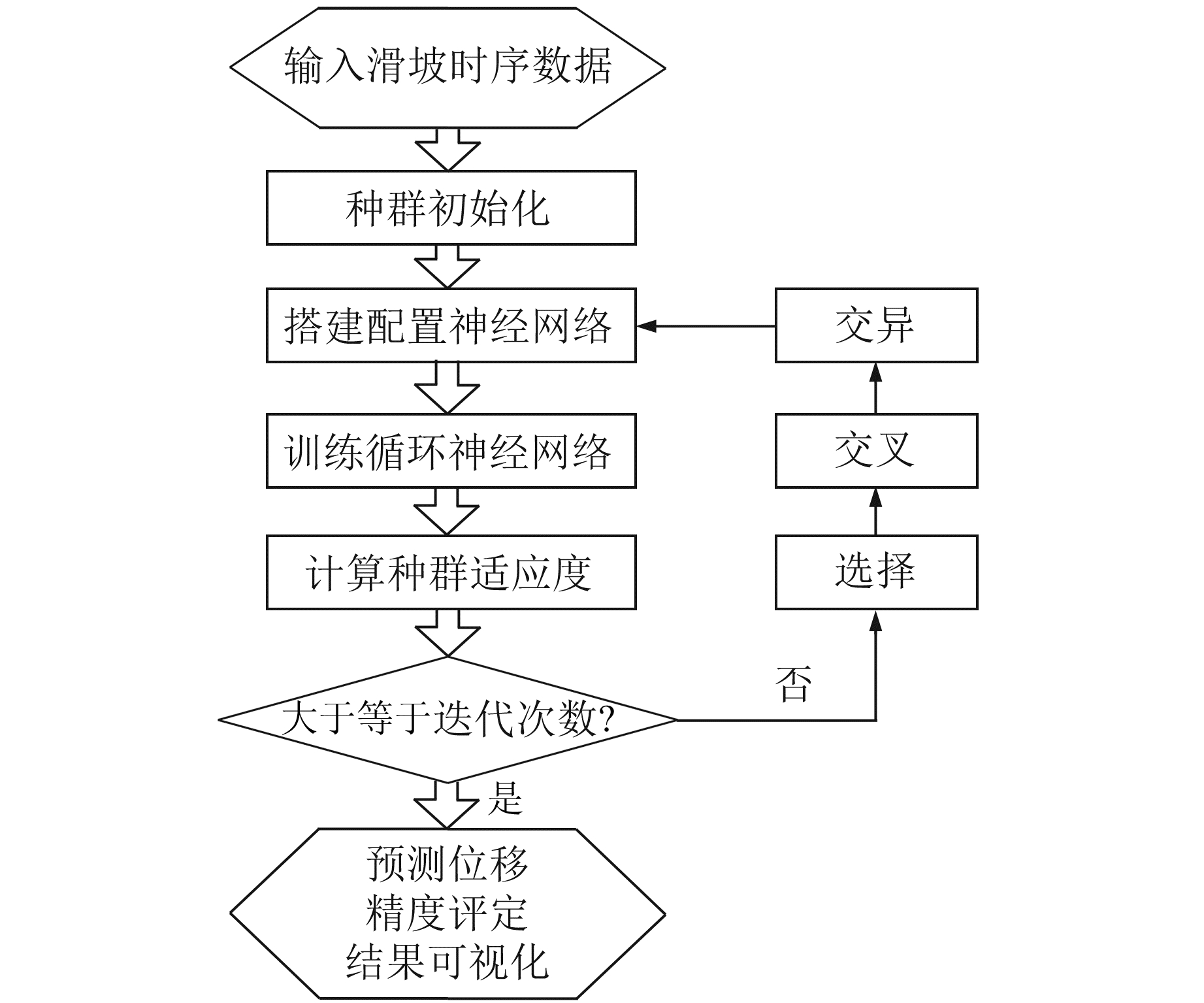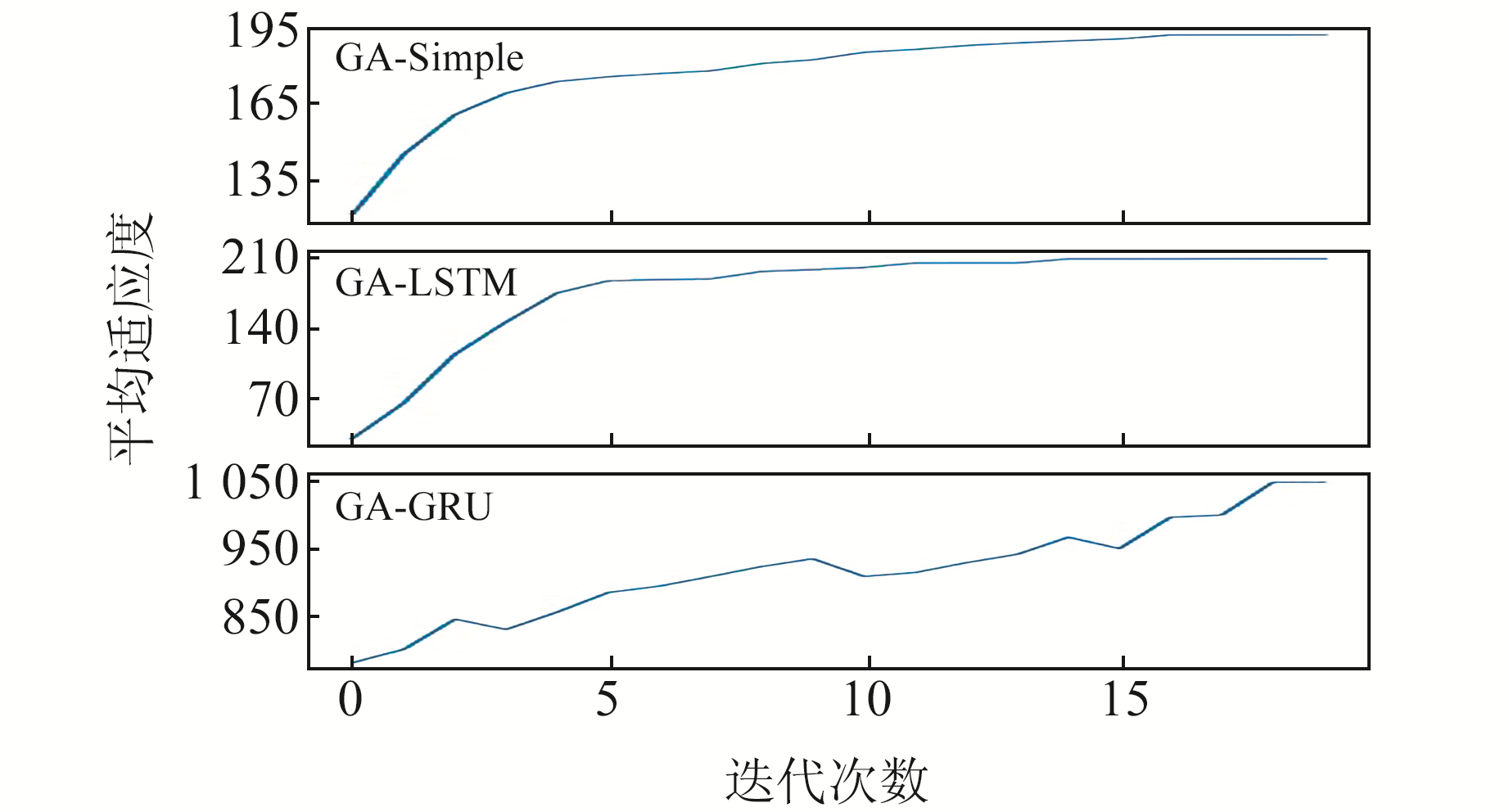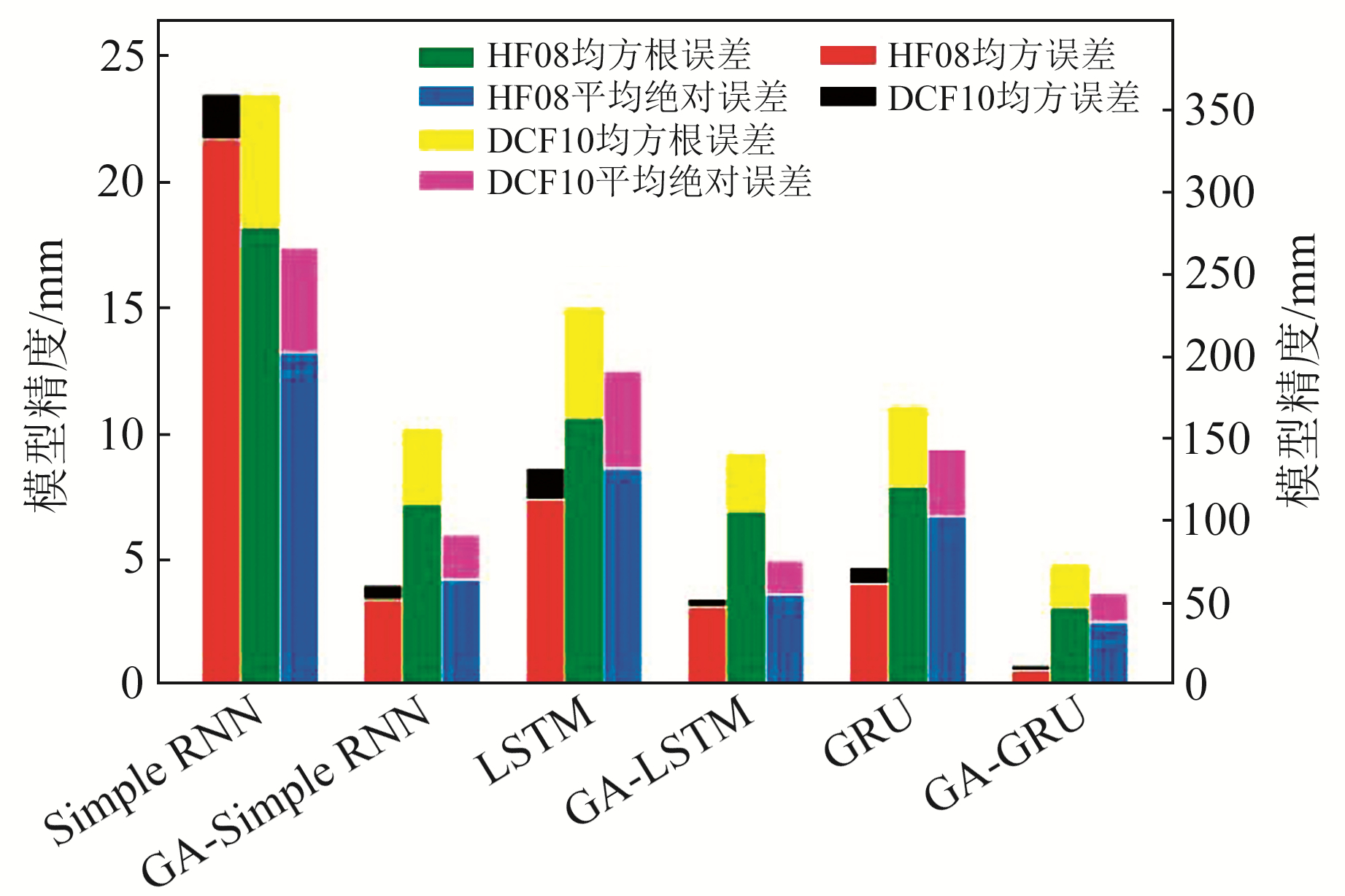﻿ 优化循环神经网络在滑坡位移预测中的应用文章快速检索 高级检索
 大地测量与地球动力学2022, Vol. 42Issue (6): 594-600  DOI: 10.14075/j.jgg.2022.06.008### 引用本文LI Lu, QU Wei, ZHANG Qin, et al. Application of Optimized Recurrent Neural Network in Prediction of Landslide Displacement[J]. Journal of Geodesy and Geodynamics, 2022, 42(6): 594-600.### Foundation support

National Natural Science Foundation of China, No.42174006, 42090055; Science Fund for Distinguished Young Scholars of Shaanxi Province, No.2022JC-18; Selection Research Projects of Science and Technology for Overseas Students in Shaanxi Province, No.13; Fundamental Research Funds for the Central Universities, No.300102261404; Science and Technology Innovation Project of Shaanxi Bureau of Surveying, Mapping and Geographic Information, No.SCK2020-03.

### Corresponding author

QU Wei, PhD, professor, PhD supervisor, majors in geological disaster high-precision geodetic monitoring and disaster mechanism, E-mail: maikerqq@163.com.

### 第一作者简介

LI Lu, postgraduate, majors in high-precision monitoring and displacement prediction of landslide disaster, E-mail: 2019126028@chd.edu.cn.

### 文章历史

1. 长安大学地质工程与测绘学院，西安市雁塔路126号，710054

1 循环神经网络及其优化模型 1.1 简单循环神经网络(SimpleRNN)

SimpleRNN是深度学习中重要的一种神经网络模型，具有可循环递归地处理滑坡历史形变数据及可对历史记忆进行建模的特殊性能，适用于解决滑坡位移时间序列预测问题。循环核中记忆体内存储着每个时刻的滑坡位移状态信息ht可表示为：

 ${h_t} = \tanh \left( {{x_t}{\mathit{\boldsymbol{w}}_{xh}} + {h_{t - 1}}{\mathit{\boldsymbol{w}}_{hh}} + {b_h}} \right)$ (1)

 ${y_t} = {\mathop{\rm softmax}\nolimits} \left( {{h_t}{\mathit{\boldsymbol{w}}_{hy}} + {b_y}} \right)$ (2)

1.2 长短期记忆网络(LSTM)

LSTM算法通过门控单元较好地解决了SimpleRNN长时间序列预测精度低的问题。LSTM的门机制可以对滑坡位移信息的流通和损失进行控制，使网络更适合于滑坡时序位移预测。LSTM算法中引入了输入门it、遗忘门ft、输出门ot、细胞态Ct、记忆体ht及候选态${\tilde C_t}$。LSTM计算过程可概括为：

 ${输入门}:{i_t} = \sigma \left( {{\mathit{\boldsymbol{W}}_i} \cdot \left[ {{h_{t - 1}}, {x_t}} \right] + {b_i}} \right)$ (3)
 ${遗忘门}:{f_t} = \sigma \left( {{\mathit{\boldsymbol{W}}_f}} \right. \cdot \left. {\left[ {{h_{t - 1}}, {x_t}} \right] + {b_f}} \right)$ (4)
 ${{输出门}}:{o_t} = \sigma \left( {{\mathit{\boldsymbol{W}}_o}.\left[ {{h_{t - 1}}, {x_t}} \right) + {b_o}} \right)$ (5)
 ${细胞态}:{C_t} = {f_t} \cdot {C_{t - 1}} + {i_t} \cdot {\tilde C_t}$ (6)
 ${记忆体}:{h_t} = {o_t} \cdot \tanh \left( {{C_t}} \right)$ (7)
 ${候选态}:{\tilde C_t} = \tanh \left( {{\mathit{\boldsymbol{W}}_c} \cdot \left[ {{h_{t - 1}}, {x_t}} \right) + {b_c}} \right.$ (8)

1.3 门控循环单元(GRU)

GRU算法将LSTM中的遗忘门与输入门合二为一，形成“更新门”。相比于LSTM模型，GRU模型更加精简。GRU使记忆体ht融合了长期滑坡位移信息和短期滑坡位移信息，其中ht包含过去滑坡位移信息ht－1和现在滑坡位移信息${\tilde h_t}$，由更新门zt分配权重，更新门和重置门rt的取值范围为0~1。GRU的计算过程可概述为：

 ${更新门}:{z_t} = \sigma \left( {{\mathit{\boldsymbol{W}}_z} \cdot \left[ {{h_{t - 1}}, {x_t}} \right]} \right)$ (9)
 ${重置门}:{r_t} = \sigma \left( {{\mathit{\boldsymbol{W}}_r} \cdot \left[ {{h_{t - 1}}, {x_t}} \right]} \right)$ (10)
 ${记忆体}:{h_t} = \left( {1 - {z_t}} \right) \cdot {h_{t - 1}} + {z_t} \cdot {\tilde h_t}$ (11)
 ${候选隐藏层}:{\tilde h_t} = \tanh \left( {\mathit{\boldsymbol{W}}.\left[ {{r_t}*{h_{t - 1}}, {x_t}} \right]} \right)$ (12)

1.4 遗传算法(GA)及其优化的循环神经网络图 1 基于遗传算法优化循环神经网络技术路线 Fig. 1 Technology road map of recurrent neural network optimized by genetic algorithm

1) 产生初始种群。设置种群规模、交叉概率、变异概率与进化代数。随机产生初始种群，每个个体基因编码为[PN1N2N3]，其中P表示神经网络层数，范围为1~3；N1N2N3分别代表第1层、第2层和第3层记忆体数量。

2) 计算适应度。适应度函数设计为均方误差的倒数乘以常系数k，这样就将寻求均方误差最小的目标转化为搜索适应度最高的个体，即最佳网络结构参数。根据个体基因编码配置循环神经网络的网络结构参数，训练模型并进行预测。根据预测结果计算第i组网络结构参数的适应度fi

 $f_{i}=k m / \sum\limits_{i=1}^{m}\left(y_{i}-\tilde{y}_{i}\right)^{2}$ (13)

3) 选择。该运算利用每组网络结构参数的适应度占种群适应度总和的比重决定其遗传到下一代的可能性。若设种群数为n，第i组网络结构参数的适应度为fi，该组参数在进化中遗传的概率pi可表示为：

 $p_{i}=f_{i} / \sum\limits_{j=1}^{n} f_{j}$ (14)

4) 交叉。以2组网络结构参数为一对，将种群中所有网络结构参数组合随机分成若干对。每对参数组合给定一个0~1的随机数p0，在给定交叉概率pc的情况下，若p0 < pc，则单点交叉产生新的网络结构参数组合，否则按照之前的网络结构参数遗传到子代。

5) 变异。如果只进行交叉操作，适应度较高的某组网络结构参数可能会造成算法过早收敛而陷入局部最优解。因此，需要对少量参数组合进行变异操作从而产生新的网络结构参数组合。每组网络结构参数给定一个0~1的随机数p1，在给定变异概率pm的条件下，如果p1 < pm则进行变异操作。

6) 逐代进化。循环计算适应度，由交叉和变异操作产生新的网络结构参数组合，返回到步骤2)。进化结束时，种群中适应度最大的参数组合即为循环神经网络的最佳参数组合。

2 滑坡时序位移预测实例 2.1 示范实验区滑坡概况与监测信息HF**为北斗监测站,DCF**为位移计监测站, 白色虚线框代表党川6 # 典型滑坡体 图 2 黑方台党川6#典型滑坡区域监测站点分布 Fig. 2 Distribution of monitoring stations of 6# typical landslide in Dangchuan, Heifangtai
2.2 循环神经网络位移预测分析图 3 3种循环神经网络预测模型预测值与观测值对比 Fig. 3 Comparison of prediction and observation results of the three kinds of recurrent neural network models表 1 3种循环神经网络对HF08北斗与DCF10位移计位移时序预测精度对比 Tab. 1 Comparison of prediction accuracy of the three kinds of recurrent neural networks in HF08 BDS and DCF10 displacement meters
2.3 基于GA优化的循环神经网络高精度位移预测分析图 4 基于遗传算法改进的3种循环神经网络预测模型种群平均适应度变化曲线 Fig. 4 Variation curves of population average fitness based on the three types of recurrent neural network models optimized by genetic algorithm图 5 基于遗传算法优化后3种循环神经网络预测模型预测值与观测值对比 Fig. 5 Comparison of prediction and observation results of the three kinds of recurrent neural network models optimized by genetic algorithm表 2 基于遗传算法优化后3种循环神经网络模型对时间序列的预测精度对比 Tab. 2 Comparison of prediction accuracy of the three kinds of recurrent neural networks optimized by genetic algorithm in HF08 BDS and DCF10 displacement meters图 6 遗传算法优化前后的循环神经网络预测模型对比 Fig. 6 Comparison chart of recurrent neural network prediction models before and after genetic algorithm optimization
3 结语

1) 遗传算法可对循环神经网络层数及每层网络记忆体数量进行随机搜索且自动确定出最佳参数组合，从而优化循环神经网络结构，显著提高网络预测性能。

2) 3种基于遗传算法改进后的循环神经网络预测模型的预测均方误差、均方根误差、平均绝对误差均较改进前循环神经网络预测精度更高，特别是GA-GRU模型预测精度最优，更加适用于滑坡长时间时序位移的高精度预测。

  佘雅文, 付广裕. 基于循环神经网络的重力异常数据推估研究[J]. 大地测量与地球动力学, 2021, 41(3): 234-237 (She Yawen, Fu Guangyu. Estimation of Gravity Anomaly Data Based on Recurrent Neural Network[J]. Journal of Geodesy and Geodynamics, 2021, 41(3): 234-237) (0)  曹国清, 张晓明, 陈亚峰. 基于PCA-LSTM的多变量矿山排土场滑坡预警研究[J]. 计算机系统应用, 2018, 27(11): 252-258 (Cao Guoqing, Zhang Xiaoming, Chen Yafeng. Early Warning of Landslide in Mined Mine Dumping Site Based on PCA-LSTM[J]. Computer Systems and Applications, 2018, 27(11): 252-258) (0)  Liu Z Q, Guo D, Lacasse S, et al. Algorithms for Intelligent Prediction of Landslide Displacements[J]. Journal of Zhejiang University-Science A: Applied Physics and Engineering, 2020, 21(6): 412-429 (0)  冯非凡, 武雪玲, 牛瑞卿, 等. 一种V/S和LSTM结合的滑坡变形分析方法[J]. 武汉大学学报: 信息科学版, 2019, 44(5): 784-790 (Feng Feifan, Wu Xueling, Niu Ruiqing, et al. A Landslide Deformation Analysis Method Using V/S and LSTM[J]. Geomatics and Information Science of Wuhan University, 2019, 44(5): 784-790) (0)  梁阳, 肖婷, 胡程, 等. 基于长期监测数据与LSTM网络的滑坡位移预测[EB/OL]. http://kns.cnki.net/kcms/detail/11.2406.TN.20210512.1045.032.html, 2021-09-21 (Liang Yang, Xiao Ting, Hu Cheng, et al. Prediction of Landslide Displacement Based on Long Term Monitoring Data and LSTM Network[EB/OL]. http://kns.cnki.net/kcms/detail/11.2406.TN.20210512.1045.032.html, 2021-09-21) (0)  王智伟, 王利, 韩清清, 等. 一种位移传感器数据解码方法及其在滑坡监测中的应用[J]. 大地测量与地球动力学, 2020, 40(4): 436-440 (Wang Zhiwei, Wang Li, Han Qingqing, et al. Data Decoding Method of the Displacement Sensor and Its Application in Landslide Monitoring[J]. Journal of Geodesy and Geodynamics, 2020, 40(4): 436-440) (0)  Pearlmutter B A. Learning State Space Trajectories in Recurrent Neural Networks[J]. Neural Computation, 1989, 1(2): 263-269 DOI:10.1162/neco.1989.1.2.263 (0)  Kim D S, Chae Y S, Kim Y S, et al. A Comparative Study between BPNN and RNN on the Settlement Prediction during Soft Ground Embankment[J]. Journal of the Society of Disaster Information, 2007, 3(1): 37-53 (0)  Hochreiter S, Schmidhuber J. Long Short-Term Memory[J]. Neural Computation, 1997, 9(8): 1 735-1 780 DOI:10.1162/neco.1997.9.8.1735 (0)  杨背背, 殷坤龙, 杜娟. 基于时间序列与长短时记忆网络的滑坡位移动态预测模型[J]. 岩石力学与工程学报, 2018, 37(10): 2 334-2 343 (Yang Beibei, Yin Kunlong, Du Juan. A Model for Predicting Landslide Displacement Based on Time Series and Long and Short Term Memory Neural Network[J]. Chinese Journal of Rock Mechanics and Engineering, 2018, 37(10): 2 334-2 343) (0)  Kurochkin I I, Volkov S S. Using GRU Based Deep Neural Network for Intrusion Detection in Software-Defined Networks[C]. IOP Conference Series: Materials Science and Engineering, Russia, 2020 (0)  栾晓东, 底青云, 雷达. 基于牛顿迭代法和遗传算法的CSAMT近场校正[J]. 地球物理学报, 2018, 61(10): 4 148-4 159 (Luan Xiaodong, Di Qingyun, Lei Da. Near-Field Correction of CSAMT Data Based on Newton Iteration Method and GA Method[J]. Chinese Journal of Geophysics, 2018, 61(10): 4 148-4 159) (0)  李天磊. 地下水诱发黄土滑坡机理及其稳定性分析[D]. 北京: 中国地质大学, 2018 (Li Tianlei. Mechanism and Stability Analysis of Loess Landslide Induced by Groundwater[D]. Beijing: China University of Geosciences, 2018) (0)  白正伟, 张勤, 黄观文, 等. "轻终端+行业云"的实时北斗滑坡监测技术[J]. 测绘学报, 2019, 48(11): 1 424-1 429 (Bai Zhengwei, Zhang Qin, Huang Guanwen, et al. Real-Time Beidou Landslide Monitoring Technology of "Light Terminal Plus Industry Cloud"[J]. Acta Geodaetica et Cartographica Sinica, 2019, 48(11): 1 424-1 429) (0)  陈玉萍, 袁志强, 周博, 等. 遗传算法优化BP网络在滑坡灾害预测中的应用研究[J]. 水文地质工程地质, 2012, 39(1): 114-119 (Chen Yuping, Yuan Zhiqiang, Zhou Bo, et al. Application of Back Propagation Neural Networks with Optimization of Genetic Algorithms to Landslide Hazard Prediction[J]. Hydrogeology and Engineering Geology, 2012, 39(1): 114-119) (0)
Application of Optimized Recurrent Neural Network in Prediction of Landslide Displacement
LI Lu1QU Wei1ZHANG Qin1     LI Jiuyuan1     WANG Yuhao1     LIU Xiangbin1
1. College of Geological Engineering and Geomatics, Chang'an University, 126 Yanta Road, Xi'an 710054, China
Abstract: We select the Heifangtai Dangchuan #6 landslide body in Gansu province as the study region, which is a typical loess landslide area in Chinese mainland. Three recurrent neural network prediction models of landslide are established using the deep learning framework Tensorflow based on the monitoring data of Beidou and displacement meter, namely, the simple recurrent neural network(SimpleRNN), long short-term memory(LSTM), and gated recurrent unit(GRU). Further, in view of the prominent problems of large subjective impact and low computational efficiency caused by the fact that the parameters of the recurrent neural network are mostly adjusted manually by experience or the grid search method, we introduce a genetic algorithm(GA) to optimize the automatic optimization selection of the parameters of the recurrent neural network. Thereby, three recurrent neural network prediction models of landslide optimized by GA are established, namely, GA-SimpleRNN, GA-LSTM, and GA-GRU. The results show that the improved three recurrent neural network prediction models with automatic optimization of parameters have better prediction performance. The GA-GRU model has the highest prediction accuracy, which is more suitable for the high-precision prediction of the long-time displacement of landslides.
Key words: landslide displacement prediction; simple recurrent neural networks(SimpleRNN); long short-term memory(LSTM); gated recurrent unit(GRU); genetic algorithm(GA)